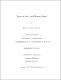## Topics in finite graph Ramsey theory##### Date
2008-01-18T15:05:39Z
##### Authors
Borgersen, Robert David
##### Abstract
For a positive integer $r$ and graphs $F$, $G$, and $H$, the graph Ramsey arrow notation $F \longrightarrow (G)^H_r$ means that for every $r$-colouring of the subgraphs of $F$ isomorphic to $H$, there exists a subgraph $G'$ of $F$ isomorphic to $G$ such that all the subgraphs of $G'$ isomorphic to $H$ are coloured the same. Graph Ramsey theory is the study of the graph Ramsey arrow and related arrow notations for other kinds of graphs" (\emph{e.g.}, ordered graphs, or hypergraphs). This thesis surveys finite graph Ramsey theory, that is, when all structures are finite. One aspect surveyed here is determining for which $G$, $H$, and $r$, there exists an $F$ such that $F \longrightarrow (G)^H_r$. The existence of such an $F$ is guaranteed when $H$ is complete, whether subgraph" means weak or induced, and existence results are also surveyed when $H$ is non-complete. When such an $F$ exists, other aspects are surveyed, such as determining the order of the smallest such $F$, finding such an $F$ in some restricted family of graphs, and describing the set of minimal such $F$'s.
##### Keywords
Ramsey, graph, Ramsey theory, graph theory, Ramsey's theorem, Ramsey numbers, graph Ramsey, induced graph Ramsey, extremal graph, Ramsey graph, linear Ramsey, restricted Ramsey, Ramsey minimal, minimal Ramsey, Ramsey arrow